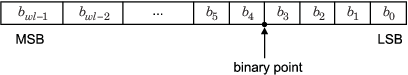## Fixed-Point Data Types

In digital hardware, numbers are stored in binary words. A binary word is a fixed-length sequence of bits (1s and 0s). How hardware components or software functions interpret this sequence of 1s and 0s is defined by the data type. Binary numbers are represented as either fixed-point or floating-point data types.

A fixed-point data type is characterized by the word length in bits, the position of the binary point, and whether it is signed or unsigned. The position of the binary point is the means by which fixed-point values are scaled and interpreted.

For example, a binary representation of a generalized fixed-point number (either signed or unsigned) is shown below:where

• bi is the ith binary digit.

• wl is the word length in bits.

• bwl-1 is the location of the most significant, or highest, bit (MSB).

• b0 is the location of the least significant, or lowest, bit (LSB).

• The binary point is shown four places to the left of the LSB. In this example, the number is said to have four fractional bits, or a fraction length of four.

Fixed-point data types can be either signed or unsigned. Whether a fixed-point value is signed or unsigned is usually not encoded explicitly within the binary word; that is, there is no sign bit. Instead, the sign information is implicitly defined within the computer architecture.

Signed binary fixed-point numbers are typically represented in computer hardware in one of three ways:

• Sign/magnitude – One bit of a binary word is always the dedicated sign bit, while the remaining bits of the word encode the magnitude of the number. Negation using sign/magnitude representation consists of flipping the sign bit from 0 (positive) to 1 (negative), or from 1 to 0.

• One's complement – Negating a binary number in one's complement requires a bitwise complement. That is, all 0s are flipped to 1s and all 1s are flipped to 0s. In one's complement notation there are two ways to represent zero. A binary word of all 0s represents "positive" zero, while a binary word of all 1s represents "negative" zero.

• Two's complement – Negation using signed two's complement representation consists of a bit inversion (translation into one's complement) followed by the binary addition of a one. For example, the two's complement of 000101 is 111011.

Two's complement is the most common representation of signed fixed-point numbers and is the only representation used by Fixed-Point Designer™ documentation.